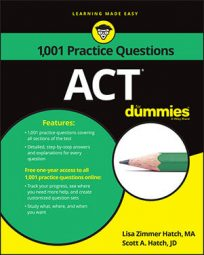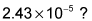##### ACTIf working with a lot of zeroes and decimals makes you feel faint, then you’d better bring some smelling salts on test day, because the ACT Math exam contains a lot of questions where you have to work with decimals.

The following practice questions will test your skills (and mettle) at converting a number from scientific notation to decimal form, and finding the difference between two decimal numbers so that you can compare the results.

## Practice questions

1. The value of 3 – 0.5 is how many times the value of 1 – 0.5?

A. 2 B. 4.5 C. 5 D. 5.5 E. 6

2. Which of the following is equal toA. 2,430 B. 243 C. 0.0243 D. 0.000243 E. 0.0000243